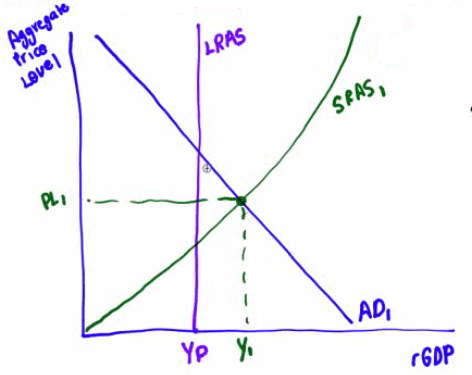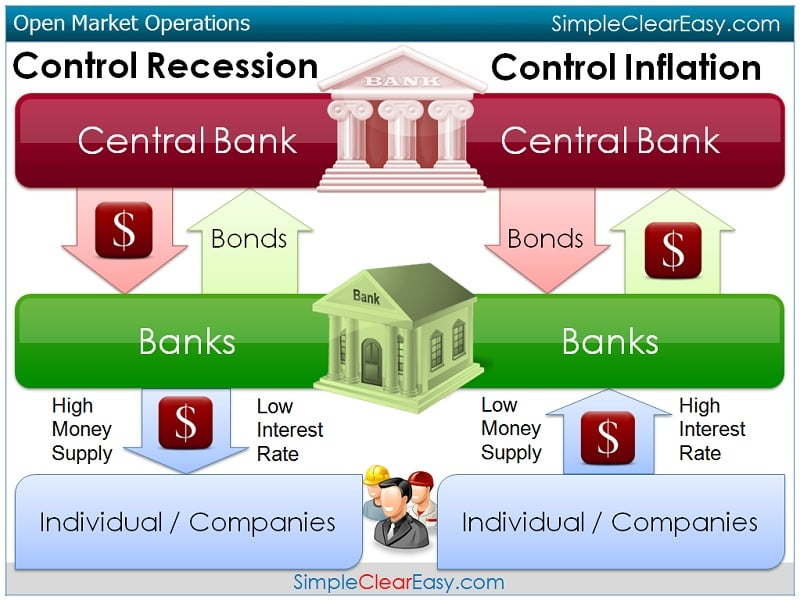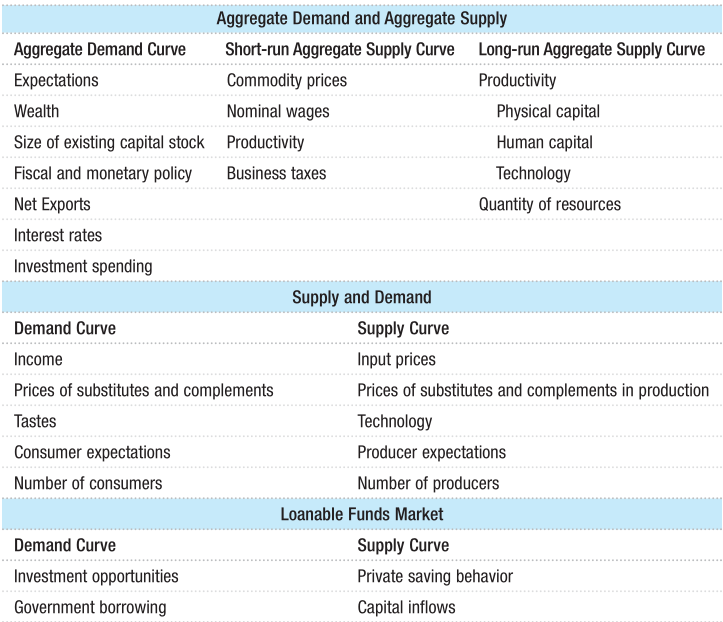# The Scenario

• Assume the US economy is operating at an aggregate output level above potential output.

• Draw a graph showing AD, SRAS, LRAS, equilibrium output & aggregate price level.

• Now assume the Fed uses contractionary monetary policy.

• Identify the open-market operation the Fed would use and draw a money market graph to show the effect of monetary policy on the nominal interest rate.

• Show how the Fed's actions will affect equilibrium in the aggregate demand and supply graph you drew previously and the new aggregate price level.

• Draw a graph of the foreign exchange market for the US dollar relative to the Euro

• How will the Fed's contractionary monetary policy affect the real interest rate in the US?

# The Inflationary Gap

• Aggregate output level above potential output: inflationary gap

• Axes

• x-axis: Y = rGDP (real GDP)

• y-axis: Aggregate Price Level

• Aggregate Demand

• Consumer Expenditures + Business Investment + Government Expenditures + Net Exports

• Y = C + I +G + NX

• SRAS

• Up-ward sloping curve
• Equilibrium output & aggregate price level

• The intersection of AD and SRAS
• LRAS

• To the left of the equilibrium

• Aggregate output level is above the potential output

• Potential output (Yp)

• The intersection of LRAS and x-axis# Money Market

Expansionary Monetary Policy Contractionary Money Policy
• Lower Discount Rate

• Raise Discount Rate

• Lower RRR

• Raise RRR

• Buy Government Securities After Lowering Target Fed Funds Rate
(Open Market Operation)

• Sell Government Securities After Raising of Target Fed Funds Rate
(Open Market Operation)• Axes

• x-axis: Quantity of Money

• y-axis: Nominal Interest Rate (r)

• Money Demand

• Downward sloping curve
• Money Supply

• Straight Vertical Line, since the Fed control the money supply

• Expansionary: MS shifts to the right, lowing the nominal interest rate

• Contractionary: MS shifts to the left, raising the nominal interest rate# New Equilibrium

• Higher interest rate = Decreased investment

• When interest rates go up, both consumption and investment decrease.

• Y↓ = C↓ + I↓ + G +NX

• Aggregate Demand↓

• Aggregate Price as a result of contractionary monetary policy# Exchange Market

• Axes

• x-axis: Quantity of Dollars

• y-axis: Euros per Dollar

• No effect in the long-run.

• Since money is neutral, monetary has a short-run but not a long-run impact

• Price level↓ Demand for US dollar↑

• Dollar has appreciated, because demand for US dollars has increased# Major Factors that Shift Curves in Each Model